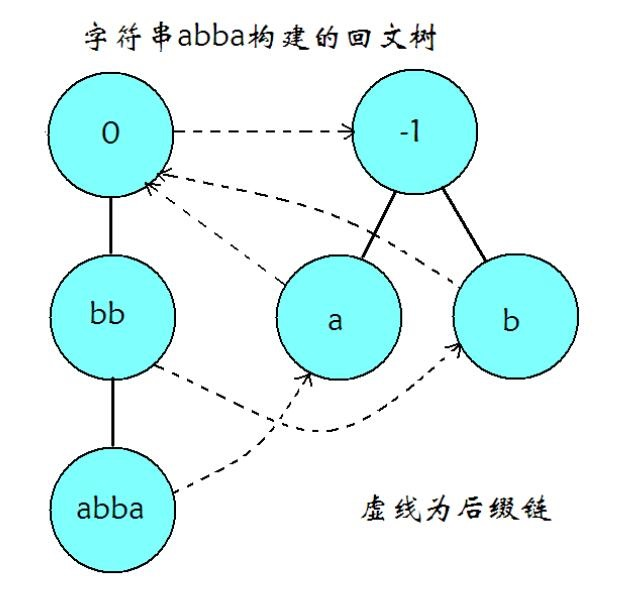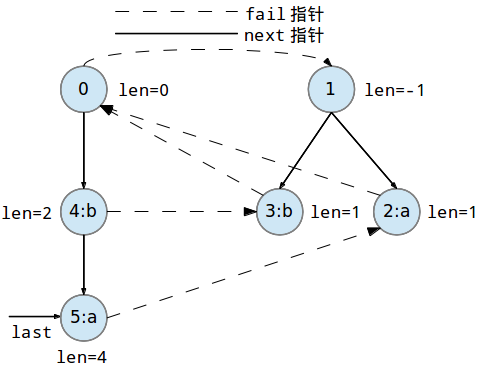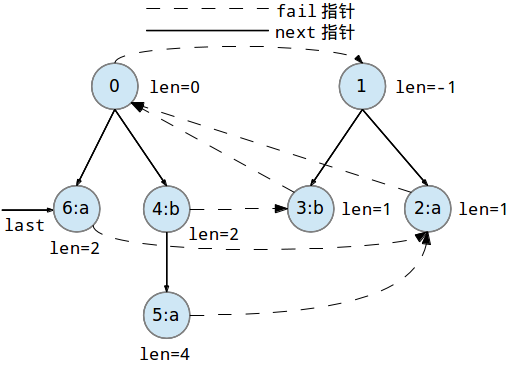# 回文自动机原理与实现# 结构## 回文树

### 节点信息

• 每个节点上记录对应回文串的长度$\mathrm{len}(u)$，若节点$v$是节点$u$转移而来的，则有$\mathrm{len}(v) = \mathrm{len}(v) + 2$。为了方便，我们规定，偶回文树树根$\mathrm{len}(rt_0)=0$，奇回文树树根$\mathrm{len}(rt_1)=-1$。
abba的PAM中，节点$u$代表串bb，节点$v$代表串abba，存在$u \rightarrow v$的转移边，$\mathrm{len}(u)=2$且$\mathrm{len}(v)=4$。
• 每个节点记录该节点对应回文串的出现次数$\mathrm{siz}(u)$。在回文树中，除了根节点以外，所有节点满足$\mathrm{siz}(u)>0$。

## 后缀链接

• $u$的后缀链接指向$v$，记作$\mathrm{fail}(u)=v$，当且仅当$v$对应的回文串是$u$对应的回文串的一个后缀，且不存在其他的$v’$，使得$\mathrm{len}(v’) > \mathrm{len}(v)$且满足上一句话的性质。这里的后缀链接的意义与AC自动机、KMP匹配算法的fail数组意义很像。
例如，对于abba的PAM，对应abba的节点$u$与对应a的节点$v$，存在$\mathrm{fail}(u)=v$。
• 为了方便，我们规定，偶回文树树根的后缀链接指向奇回文树树根，即$\mathrm{fail}(rt_0)=rt_1$。
• 后缀链接构成了一棵以奇回文树树根为根的有根树，方向从叶子指向根。

# 构造

## 流程

PAM的构造法在字符集为常数且较小时，是线性复杂度的。与SAM相似的是，PAM的构造法也是一个增量算法，因此，应该解释为，每次在母串后插入一个字符，更新PAM的复杂度是$O(1)$的。
PAM中，我们需要记下上一次插入的节点位置，即$lst$。1. 从上一次插入的节点位置$lst$开始沿$\mathrm{fail}(u)$上跳，找到第一个节点，使得该节点对应的一个母串的后缀后加上插入的字符$c$构造出了一个新的回文后缀。
在本例中，abba后加入字符a构成的新串是abbaa，这一过程实际上是找到一个新串的一个最长回文后缀，也就是aa的父节点，我们找到的是偶回文树的树根$0$。
2. 若查询到该回文串已存在节点，则对该节点$\mathrm{siz}(u)$加1。
3. 若不存在该回文串的节点，则在父节点下新建节点，并采用与1相似的过程，从父节点沿$\mathrm{fail}(u)$上跳，直到找到一个第一个最长的回文后缀，从而计算出该节点的$\mathrm{fail}(u)$值。
在本例中，aa回文串的最长回文后缀是a，容易发现，从父节点$0$开始上跳，会跳到奇回文树根$1$，从而找到对应的最长回文后缀节点$2$，即对于新加点$u=6$，有$\mathrm{fail}(6)=2$。
4. 更新PAM的$lst$值及刚才插入的回文后缀的$\mathrm{siz}(u)$值。